# Root

Use law of square roots roots:

$\sqrt{\sqrt{\sqrt{6}}}=\sqrt[n]{6}$

n =  24

### Step-by-step explanation: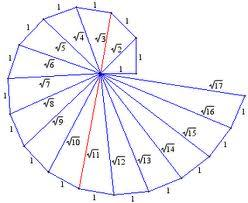Did you find an error or inaccuracy? Feel free to write us. Thank you!## Related math problems and questions:

• Square roots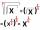What is equal to the product of the square roots of 295936?
• Cube root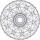Find cube root of 18
• CubesOne cube is an inscribed sphere and the other one described. Calculate the difference of volumes of cubes, if the difference of surfaces in 257 mm2.
• HoleIn the center of the cube with edge 14 cm we will drill cylinder shape hole. Volume of the hole must be 27% of the cube. What drill diameter should be chosen?
• Cube in a sphereThe cube is inscribed in a sphere with a volume 7253 cm3. Determine the length of the edges of a cube.
• Square 2Points D[10,-8] and B[4,5] are opposed vertices of the square ABCD. Calculate area of the square ABCD.
• Cube zoomHow many percents do we increase the volume and surface of the cube if we magnify its edge by 38 %?
• Roots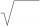Calculate the square root of these numbers:
• SquarePoints A[9,9] and B[-4,1] are adjacent vertices of the square ABCD. Calculate the area of the square ABCD.
• Cube - wall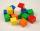V kocke ABCDEFGH je ?. Aký je povrch kocky?
• Area of RTCalculate the right triangle area that hypotenuse has length 14, and one hypotenuse segment has length 5.
• Pyramid roof2/4 of the area of ​​the roof-shaped regular tetrahedral pyramid with base edge 10 m and height of 4 m is already covered with roofing. How many square meters still need to be covered?
• Digits A, B, CFor the various digits A, B, C is true: the square root of the BC is equal to the A and sum B+C is equal to A. Calculate A + 2B + 3C. (BC is a two-digit number, not a product).
• Cube diagonalDetermine the length of the cube diagonal with edge 37 mm.
• Geometric mean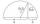Calculate the geometric mean of numbers a=15.2 and b=25.6. Determine the mean by construction where a and b are the length of the lines.
• Triangle ABCCalculate the sides of triangle ABC with area 1404 cm2 and if a: b: c = 12:7:18Determine the numbers b, c that the numbers x1 = -1 and x2 = 3 were roots of quadratic equation: -3x 2 + b x + c = 0